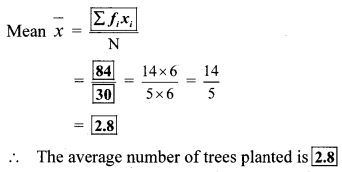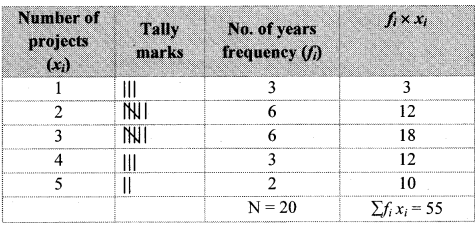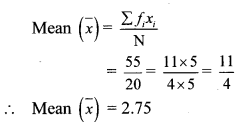Maharashtra Board Class 8 Maths Solutions Chapter 11 Statistics Practice Set 11.1

Maharashtra State Board Class 8 Maths Solutions Chapter 11 Statistics Practice Set 11.1

Question 1.
The following table shows the number of saplings planted by 30 students. Fill in the boxes and find the average number of saplings planted by each student.

 No. of saplings (Scores) xi No. of students (frequency) fi fi × xi 1 4 4 2 6 __ 3 12 __ 4 8 __ N = __ ∑ fi × xi = __Solution:

 No. of saplings (Scores) xi No. of students (frequency) fi fi × xi 1 4 4 2 6 12 3 12 36 4 8 32 N = __ ∑ fi × xi = 84Question 2.
The following table shows the electricity (in units) used by 25 families of Eklara village in a month of May. Complete the table and answer the following questions.

 Electricity used (Units) xi No. of families (frequency) fi fi × xi 30 4 45 6 60 12 75 8 90 3 N = __ ∑ fi × xi =

i. How many families use 45 units electricity?
ii. State the score, the frequency of which is 5.
iii. Find N, and ∑ fi × xi .
iv. Find the mean of electricity used by each family in the month of May.
Solution:

 Electricity used (Units) xi No. of families (frequency) fi fi × xi 30 7 210 45 2 90 60 8 480 75 5 375 90 3 270 N = 25 ∑ fi × xi = 1425

i. 2 families used 45 units of electricity.
ii. The score for which the frequency is 5 is 75
iii. N = 25 and ∑ fi × xi = 1425
iv.The mean of electricity used by each.

Question 3.
The number of members in the 40 families in Bhilar are as follows:
1, 6, 5, 4, 3, 2, 7, 2, 3, 4, 5, 6, 4, 6, 2, 3, 2, 1, 4, 5, 6, 7, 3, 4, 5, 2, 4, 3, 2, 3, 5, 5, 4, 6, 2,3, 5, 6, 4, 2. Prepare a frequency table and And the mean of members of 40 families.
Solution:∴ The mean of the members of 40 families is 3.9.

Question 4.
The number of Science and Mathematics projects submitted by Model high school, Nandpur in last 20 years at the state level science exhibition is:
2, 3 ,4, 1, 2, 3, 1, 5, 4, 2, 3, 1, 3, 5, 4, 3, 2, 2, 3, 2. Prepare a frequency table and find the mean of the data.
Solution:∴ The mean of the given data is 2.75.

Maharashtra Board Class 8 Maths Chapter 11 Statistics Practice Set 11.1 Intext Questions and Activities

Question 1.
The number of pages of a book Ninad read for five consecutive days were 60, 50, 54, 46, 50. Find the average number of pages he read everyday. (Textbook pg. no. 67)
Solution:
$$\frac{60++++50}{}=\frac{260}{}=$$
∴ Average number of pages read daily is 52